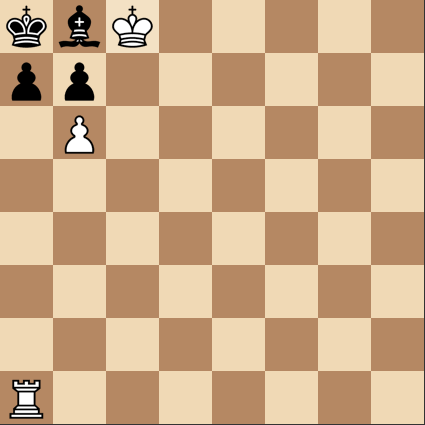Fastest checkmate 3

Logic Level 2

In the position below, how can White give the fastest checkmate?The target square of the last move can be represented as a coordinate $(x,y)$, where the bottom left corner is at $(0,0)$. Input $y$ as your answer.

×